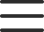# 怎样使用super关键字调用父类？super关键字用于访问和调用对象在父类上的方法，可以调用父类的构造方法，也可以调用父类的普通方法。示例代码如下。

```class Father {
constructor(x, y) {
this.x = x;
this.y = y;
}
sum() {
console.log(this.x + this.y);
}
}
class Son extends Father {
constructor(x, y) {
super(x, y); //调用父类的构造方法
}
}
var son = new Son(1, 2);
son.sum(); //输出结果：3```

super关键字也可以调用父类的普通方法，示例代码如下。

```class Father {
say() {
return '我是父类';
}
class Son extends Father {
say() {
console.log(super.say() + '的子类'）;
}
}
var son = new Son(1, 2);
son.say(); //输出结果：我是父类的子类```

```class Father {
constructor(x, y) {
this.x = x;
this.y = y;
}
sum() {
console.log(this.x + this.y);
}
}
class Son extends Father {
constructor(x, y) {
super(x, y); // super必须在子类的this之前调用
this.x = x;
this.y = y;
}
subtract() { //子类特有的方法
console.log(this.x - this.y);
}
}
var son = new Son(5, 3);
son.sum(); //输出结果：8
son.subtract(); //输出结果：2```

final关键字只能赋值一次不能多次赋值？

ES6中class关键字是怎样定义和使用类的？

static关键字怎样控制成员变量？